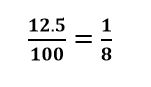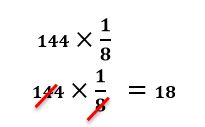# How to add percentages using fractions

Want to find out how to add percentages using fractions? We've provided a step-by-step guide of how to add percentages using fractions to teach you everything you need to know.## Step 1: Change the percentage to fraction in the form of: (percentage )/100

This means that to add 25% of 72 to it, we convert 25% to fraction first.

## Step 2: Multiply the fraction with the given base value

Cancel common factors whenever you can to make it easier. So, multiply 72 by 1/4.

## Step 3: Add the values

Add the values from Step 2 to the given base value

## Examples fn adding percentages using fractions

**Q1) Find the value if we add 12.5% to 144. **

To do so, let’s express 12.5% as (12.5)/100Multiply 144 by 1/8 and simplify the product.We can then add 18 to 144 : 144+18=162

Hence, we have 162 if we add 12.5% to 144.

#### Join today

The fastest way to practice

Unlock our complete testing platform and improve faster that ever.